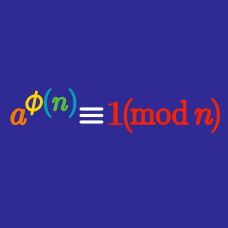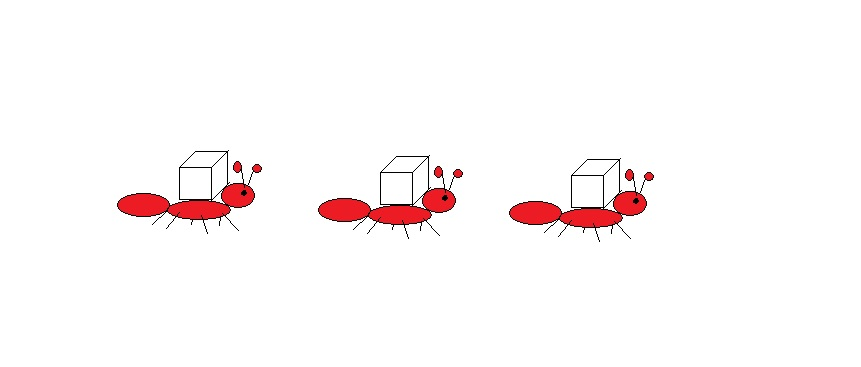Number Theory

# Euler's Theorem: Level 3 Challenges

An army of worker ants was carrying sugar cubes back into their colony. In there, the ants put 1 sugar cube into the first room, 2 into the second, 4 into the third, and doubling the amount so on until the $101^\text{th}$ room.

Then the queen ant decided to build bigger cubic blocks of $5\times 5\times 5$ sugar cubes from all they had previously collected. How many sugar cubes would remain after all these build-ups?How many positive integers $n>1$ evenly divide $a^{13}-a$ for all positive integers $a?$

Find the sum of all prime numbers $p$ such that $p|\underset { p }{ \underbrace { 111\dots 1 } }$.

$\Huge {\color{#3D99F6}9}^{{\color{#20A900}8}^{{\color{#D61F06}7}^{{\color{#624F41}6} ^{\color{magenta}5}}}}$

What are the last two digits when this integer fully expanded out?

Let $P$ be product of all positive integers less than 720 which are relatively prime to 720. What is the remainder when $P^2$ is divided by 720?

Find the tens digit of $\Large 2014^{2014^{2014}}.$

×